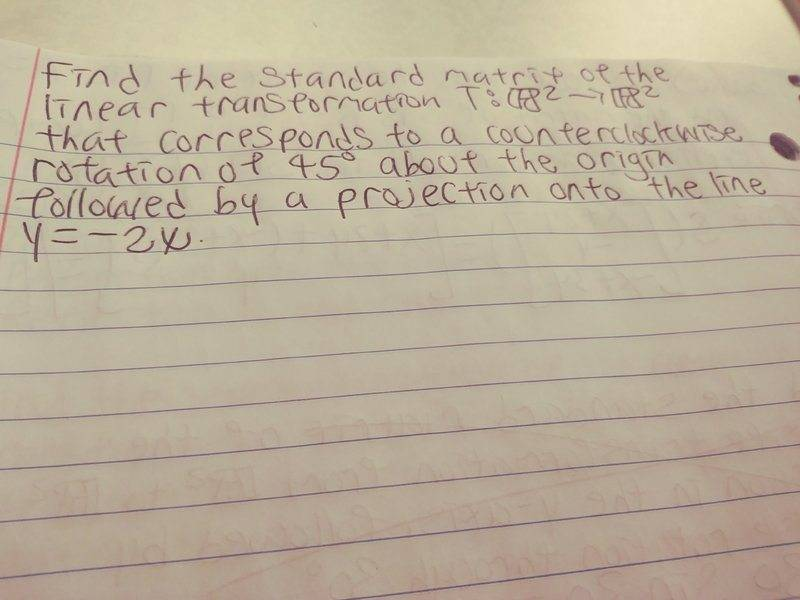# Find the standard matrix of the linear transformation

Math100

## Homework StatementNone.

## The Attempt at a Solution

I know that the standard matrix of a counterclockwise rotation by 45 degrees is:
[cos 45 -sin 45]
[sin 45 cos 45]
=[sqrt(2)/2 -sqrt(2)/2]
[sqrt(2)/2 sqrt(2)/2]
But the problem says "followed by a projection onto the line y=-2x", how do I find that?

Homework Helper
Dearly Missed

## Homework Statement

View attachment 224780

None.

## The Attempt at a Solution

I know that the standard matrix of a counterclockwise rotation by 45 degrees is:
[cos 45 -sin 45]
[sin 45 cos 45]
=[sqrt(2)/2 -sqrt(2)/2]
[sqrt(2)/2 sqrt(2)/2]
But the problem says "followed by a projection onto the line y=-2x", how do I find that?

Where does the point ##(x,y) = (a,b)## end up after the transformation?

Homework Helper
do you know about orthonormal bases?, i.e. a basis of length one vectors and mutually perpendicular? for such a basis, it is easy to expand a vector as a linear combination of the basis vectors, using dot products. And the point is that the component in the direction of say the first vector, will be the orthogonal projection onto the line spanned by that vector. to be precise, if u is any unit length vector, and v is any other vector, then |u.v| is the length of the projection of v onto the line spanned by u.

Math100
So how do I find the point end up after the transformation?